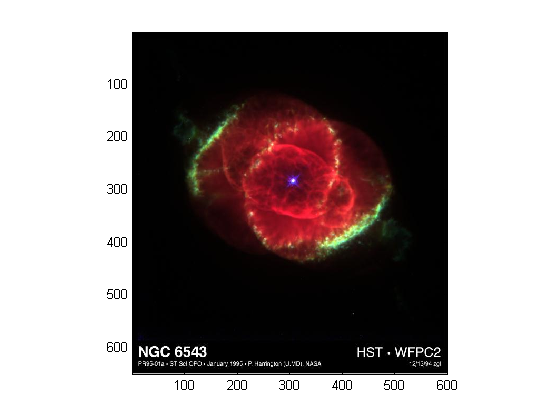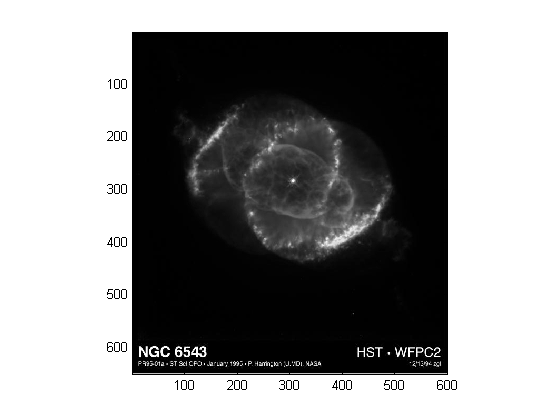## 8-Bit and 16-Bit Images

### Indexed Images

Double-precision (64-bit) floating-point numbers are the default MATLAB® representation for numeric data. However, to reduce memory requirements for working with images, you can store images as 8-bit or 16-bit unsigned integers using the numeric classes `uint8` or `uint16`, respectively. An image whose data matrix has class `uint8` is called an 8-bit image; an image whose data matrix has class `uint16` is called a 16-bit image.

The `image` function can display 8- or 16-bit images directly without converting them to double precision. However, `image` interprets matrix values slightly differently when the image matrix is `uint8` or `uint16`. The specific interpretation depends on the image type.

If the class of `X` is `uint8` or `uint16`, its values are offset by 1 before being used as colormap indices. The value 0 points to the first row of the colormap, the value 1 points to the second row, and so on. The `image` command automatically supplies the proper offset, so the display method is the same whether `X` is `double`, `uint8`, or `uint16`:

`image(X); colormap(map);`

The colormap index offset for `uint8` and `uint16` data is intended to support standard graphics file formats, which typically store image data in indexed form with a 256-entry colormap. The offset allows you to manipulate and display images of this form using the more memory-efficient `uint8` and `uint16` arrays.

Because of the offset, you must add 1 to convert a `uint8` or `uint16` indexed image to `double`. For example:

```X64 = double(X8) + 1; or X64 = double(X16) + 1;```

Conversely, subtract 1 to convert a `double` indexed image to `uint8` or `uint16`:

```X8 = uint8(X64 - 1); or X16 = uint16(X64 - 1);```

### Intensity Images

The range of `double` image arrays is usually [0, 1], but the range of 8-bit intensity images is usually [0, 255] and the range of 16-bit intensity images is usually [0, 65535]. Use the following command to display an 8-bit intensity image with a grayscale colormap:

`imagesc(I,[0 255]); colormap(gray);`

To convert an intensity image from `double` to `uint16`, first multiply by 65535:

`I16 = uint16(round(I64*65535));`

Conversely, divide by 65535 after converting a `uint16` intensity image to `double`:

`I64 = double(I16)/65535;`

### RGB Images

The color components of an 8-bit RGB image are integers in the range [0, 255] rather than floating-point values in the range [0, 1]. A pixel whose color components are (255,255,255) is displayed as white. The `image` command displays an RGB image correctly whether its class is `double`, `uint8`, or `uint16`:

`image(RGB);`

To convert an RGB image from `double` to `uint8`, first multiply by 255:

`RGB8 = uint8(round(RGB64*255));`

Conversely, divide by 255 after converting a `uint8` RGB image to `double`:

`RGB64 = double(RGB8)/255`

To convert an RGB image from `double` to `uint16`, first multiply by 65535:

`RGB16 = uint16(round(RGB64*65535));`

Conversely, divide by 65535 after converting a `uint16` RGB image to `double`:

`RGB64 = double(RGB16)/65535;`

### Mathematical Operations Support for uint8 and uint16

To use the following MATLAB functions with `uint8` and `uint16` data, first convert the data to type `double`:

For example, if `X` is a `uint8` image, cast the data to type `double`:

`fft(double(X))`

In these cases, the output is always `double`.

The `sum` function returns results in the same type as its input, but provides an option to use double precision for calculations.

#### MATLAB Integer Mathematics

See Arithmetic Operations on Integer Classes for more information on how mathematical functions work with data types that are not doubles.

Most Image Processing Toolbox™ functions accept `uint8` and `uint16` input. If you plan to do sophisticated image processing on `uin`t8 or `uint16` data, consider including that toolbox in your MATLAB computing environment.

### Other 8-Bit and 16-Bit Array Support

You can perform several other operations on `uint8` and `uint16` arrays, including:

• Reshaping, reordering, and concatenating arrays using the functions `reshape`, `cat`, `permute`, and the `[]` and `'` operators

• Saving and loading `uint8` and `uint16` arrays in MAT-files using `save` and `load`. (Remember that if you are loading or saving a graphics file format image, you must use the commands `imread` and `imwrite` instead.)

• Locating the indices of nonzero elements in `uint8` and `uint16` arrays using `find`. However, the returned array is always of class `double`.

• Relational operators

### Converting an 8-Bit RGB Image to Grayscale

You can perform arithmetic operations on integer data, which enables you to convert image types without first converting the numeric class of the image data.

This example reads an 8-bit RGB image into a MATLAB variable and converts it to a grayscale image:

```rgb_img = imread('ngc6543a.jpg'); % Load the image image(rgb_img) % Display the RGB image axis image;```Note

This image was created with the support of the Space Telescope Science Institute, operated by the Association of Universities for Research in Astronomy, Inc., from NASA contract NAs5-26555, and is reproduced with permission from AURA/STScI. Digital renditions of images produced by AURA/STScI are obtainable royalty-free. Credits: J.P. Harrington and K.J. Orkowski (University of Maryland), and NASA.

Calculate the monochrome luminance by combining the RGB values according to the NTSC standard, which applies coefficients related to the eye's sensitivity to RGB colors:

```I = .2989*rgb_img(:,:,1)... +.5870*rgb_img(:,:,2)... +.1140*rgb_img(:,:,3); ```

`I` is an intensity image with integer values ranging from a minimum of zero:

```min(I(:)) ans = 0 ```

to a maximum of 255:

```max(I(:)) ans = 255 ```

To display the image, use a grayscale colormap with 256 values. This avoids the need to scale the data-to-color mapping, which is required if you use a colormap of a different size. Use the `imagesc` function in cases where the colormap does not contain one entry for each data value.

Now display the image in a new figure using the gray colormap:

```figure; colormap(gray(256)); image(I); axis image;```#### Related Information

Other colormaps with a range of colors that vary continuously from dark to light can produce usable images. For example, try `colormap(summer(256))` for a classic oscilloscope look. See `colormap` for more choices.

The `brighten` function enables you to increase or decrease the color intensities in a colormap to compensate for computer display differences or to enhance the visibility of faint or bright regions of the image (at the expense of the opposite end of the range).

### Summary of Image Types and Numeric Classes

This table summarizes how data matrix elements are interpreted as pixel colors, depending on the image type and data class.

Image Type

double Data

uint8 or uint16 Data

Indexed

Image is an m-by-n array of integers in the range [1, p].

Colormap is a p-by-3 array of floating-point values in the range [0, 1].

Image is an m-by-n array of integers in the range [0, –1].

Colormap is a p-by-3 array of floating-point values in the range [0, 1].

Intensity

Image is an m-by-n array of floating-point values that are linearly scaled to produce colormap indices. The typical range of values is [0, 1].

Colormap is a p-by-3 array of floating-point values in the range [0, 1] and is typically grayscale.

Image is an m-by-n array of integers that are linearly scaled to produce colormap indices. The typical range of values is [0, 255] or [0, 65535].

Colormap is a p-by-3 array of floating-point values in the range [0, 1] and is typically grayscale.

RGB (Truecolor)

Image is an m-by-n-by-3 array of floating-point values in the range [0, 1].

Image is an m-by-n-by-3 array of integers in the range [0, 255] or [0, 65535].